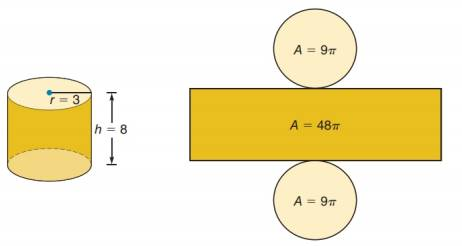Chapter A.1, Problem 32E### Elementary Geometry for College St...

6th Edition
Daniel C. Alexander + 1 other
ISBN: 9781285195698

#### Solutions

Chapter
Section### Elementary Geometry for College St...

6th Edition
Daniel C. Alexander + 1 other
ISBN: 9781285195698
Textbook Problem
1 views

# The right circular cylinder shown in the accompanying figure has circular bases that have areas of 9π square units. The side has an area of 48π square units. Find an expression for the total surface area.To determine

To find:

The expression for the total surface area of the right circular cylinder.

Explanation

The total surface area of the right circular cylinder is equal to the sum of the areas of two congruent circles and one rectangle.

Calculation:

It is given that the area of circular bases and rectangle are 9π and 48π square units...

### Still sussing out bartleby?

Check out a sample textbook solution.

See a sample solution

#### The Solution to Your Study Problems

Bartleby provides explanations to thousands of textbook problems written by our experts, many with advanced degrees!

Get Started